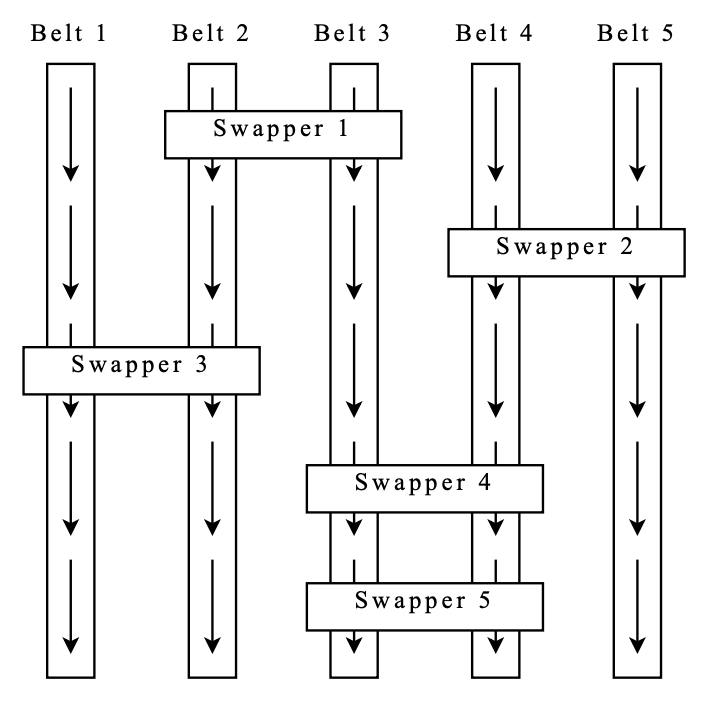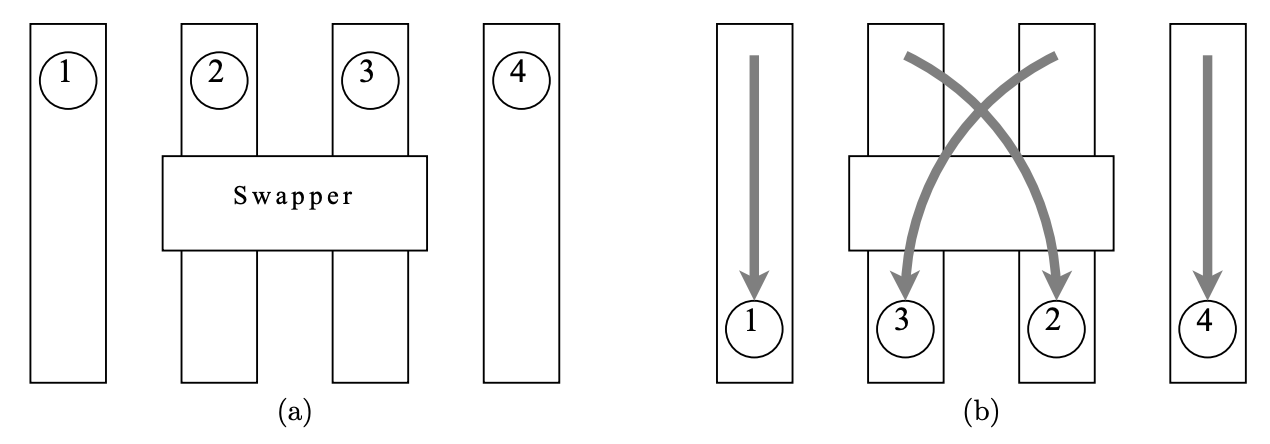시간 제한 메모리 제한 제출 정답 맞은 사람 정답 비율
2 초 512 MB 0 0 0 0.000%

## 문제

Professor X has recently revealed his latest invention to the world: the Ultimate Bead Swapper (UBS). As the name implies, it can make a sequence of beads much more interesting by swapping some beads in it!

The UBS has N conveyor belts placed in north-south direction in parallel. The conveyor belts are numbered 1 to N from left to right. Every belt moves from north to south at the same speed. There are M swappers placed between adjacent conveyors. No two swappers are equally far from the north end of the UBS. (In other words, they can be totally ordered according to how far they are from the north end.) The swappers are numbered 1 to M from north to south. Figure 1 shows the UBS when viewed from above.Figure 1: An Ultimate Bead Swapper with 5 conveyor belts and 5 swappers.

To use the UBS, N beads are placed at the north end of the conveyor belts at the same time so that they form a horizontal row as they move along the belt. When two beads come under a swapper, the bead on the right conveyor belt is moved to the left conveyor belt, and the bead on the left conveyor belt is moved to the right conveyor. After being swapped, the two beads do not break the horizontal row. Figure 2 illustrates the behavior of a swapper.Figure 2: (a) Four beads move along the conveyor belts. (b) Bead 2 and 3 trade places after going under the swapper.

Write a program that, given the number of conveyor belts N, the number of swappers M, and the positions of each swapper, answer questions of the form:

Given K and J, for the bead that is placed on Belt K at the north end of the UBS, which belt is the bead on after all beads just move past Swapper J?

## 입력

Your program should read from standard input. The first line contains the number of conveyor belts N(1 ≤ N ≤ 300, 000) and the number of swappers M(1 ≤ M ≤ 300, 000).

Swappers are listed from north to south in the following M lines. Each line contains one integer P(1 ≤ P ≤ M − 1), meaning that there is a swapper over conveyor belt P and P + 1.

## 인터랙션

After reading the input above, your program should call functions from the following library specified in Table 1. The functions must be called in the following order:

1. It calls the function getNumQuestions to retrieve Q(1 ≤ Q ≤ 300, 000), the number of questions it will be asked.
2. For Q times, it should:
1. Call the function getQuestion to receive one question.
2. Call the function answer to answer the question it just received.

We emphasize that getNumQuestions must be called first and only once. getQuestion and answer must be called alternately: after calling one getQuestion, your program may not call getQuestion until it calls answer, and vice versa. If your program violates this convention when running a test scenario, you will receive a 0% score for that test scenario.

## 구현

The source code must contain the line:

#include "beadslib.h"

Function Prototype Description
int getNumQuestions() Return the number of questions your program is going to be asked.
void getQuestion(int &K, int &J) K is set to the number of the conveyor belt that the bead is placed at the north end of the UBS.
J is set to the number of the swapper.
void answer(int x) Report that the answer to the question corresponding to the last getQuestion is x.

Table 1: Interaction library.

## 샘플 프로그램

You will be provided a zip file containing source code of sample libraries and programs. The file contains three directories — pascal, c, and cpp — for source code in Pascal, C, and C++, respectively. Each directory will contain a source code of a mock interaction library, and the source code of a program that calls the library functions in the correct order.

For C++, the prototypes of the mock library functions are also given in beadslib.h (but the file is not the same as the one for C). The functions are specified in beadslib.cpp. The file sample.cpp is the source code of the program that uses the library correctly.

The mock library behaves as follows:

• When getNumQuestions of the mock library is called, it opens the file questions.txt, reads the number of questions, and returns what is read.
• When getQuestion is called, it reads K and J from questions.txt.
• When answer is called, it prints the argument x to standard output.
• The library prints an error message to standard output every time a function is called out of the correct order.

The file questions.txt has the following format. The first line contains the number of questions Q. Each of the next Q lines contains two integers K, the number of a conveyor belt, and J, the number of a swapper.

## 예제 입력 1

5 5
2
4
1
3
3


## 예제 출력 1

2
3 4
5 5


## 인터랙션 예시

Function Call Return Value(s) and Explanation
getNumQuestions(); 2
The program will be asked two questions.
getQuestion(K, J); K=3, J=4
For the bead that is placed on Belt 3 at the north end of the UBS, which belt is the bead on after all beads just move past Swapper 4?
answer(1); After every bead passes Swapper 4, the bead in question is on Belt 1.
getQuestion(K, J); K=5, J=5
For the bead that is placed on Belt 5 at the north end of the UBS, which belt is the bead on after all beads just move past Swapper 5?
answer(4); After every bead passes Swapper 5, the bead in question is on Belt 4.

## 채점 및 기타 정보

• 예제는 채점하지 않는다.